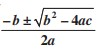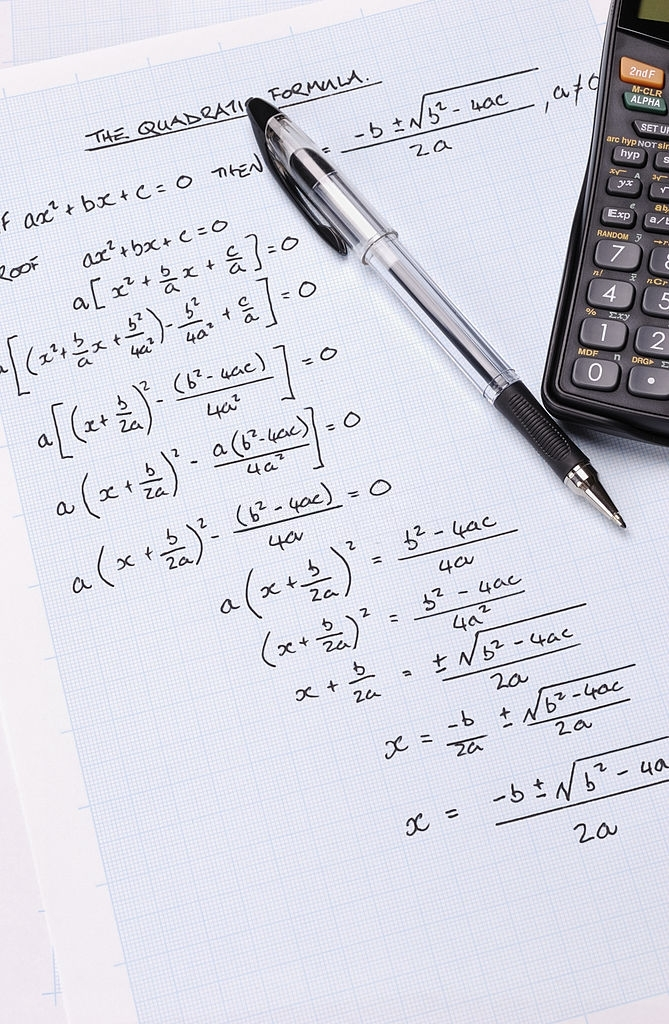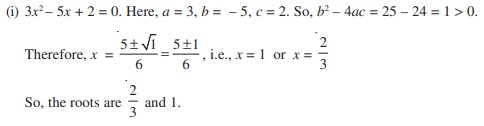# Quadratic Equations for Competitive Exams

## What is Quadratic Equation?

Quadratic  Equation can be any equation that can be arranged is the form of  ax2 + bx +c.  Here X is called Variable, a & b are called coefficient of x2 & X respectively, and C is called Constant.

Quadratic Equation Standard  Form ax2 + bx +c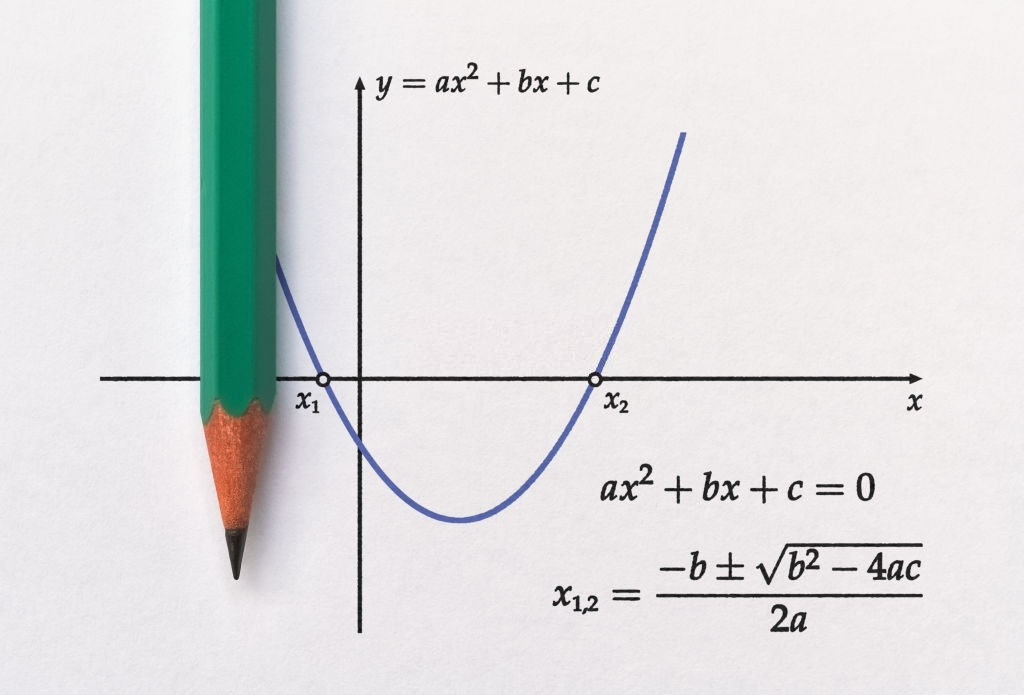## How Important is Quadratic Equations for Competitive Exams?

Quadratic Equations for Competitive Exams is an essential topic. 3-5 Questions from this topic is asked every year in each competitive exam. In Banking exams like IBPS PO, IBPS Clerk, IBPS RRB, NABARD, SBI, RBI & Other Banks individual Exams five questions are mostly asked.

## What are Roots or zeroes of Quadratic Equation?

Every quadratic equation has 2 solutions, called Roots or some times Zeroes. These Roots or Solutions may or may not be real and may or may not be Equal.

Sum and Product of roots of a quadratic equation: If α and β are the roots or zeroes of a quadratic equation, then

Sum of the Roots = α+β= -b/a = coefficient of x/coefficient of x2

Product of the Roots = αβ = c/a = constant term/coefficient of x2

## What is Discriminant or D in Quadratic Equation ?

Discriminant or D is equals to b raised to power 2 minus product of 4, a, & b.

D=b2-4ac

When the Discriminant or D (i.e b2−4ac) is:

• positive, there are 2 real solutions or roots.
• zero, there is one real solution or roots.
• negative, there are 2 complex solutions or no real roots.

## How to solve Quadratic Equation ?

In order to solve Quadratic Equation one can use any of the following five method-

2. Completing square
3. Graphing Quadratic Equation
4. The Quadratic Equation
5. Online Quadratic Equation Solver

In Factoring Quadratic Equation method you have to follow following steps.

Step 1: First multiply a & c.

Step 2: Find two Factors of the product you got in step one whose sum or diference (depend upon the sign before C) is equal to b.

Step 3: Find common in first two and last two terms

Step 4: You will notice that the equation you got in brackets are same, now take it as common and write rest terms in another bracket.

Step 5: Now put both brackets equal to zero and find both two Zeroes or Roots of the given Quadratic Equation.

For Example solve the equation x2-3x-10=0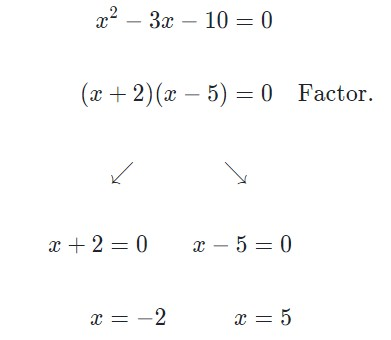Under Completing square Method follow the following steps-

Step 1: Isolate C.

Step 2: Divide all 3 terms from the coefficient of a.

Step 3: Half the coefficient of X, and add the square of it on the both side of the equation.

Step 4: Left side of the equation will become a perfect square.

Step 5: Now a single perfect square is on left side and a number on right side will left.

Step 6: Now Square root both side.

Step 7: Now find value of X.

For Example Find Roots of x2 + 10x − 4 = 0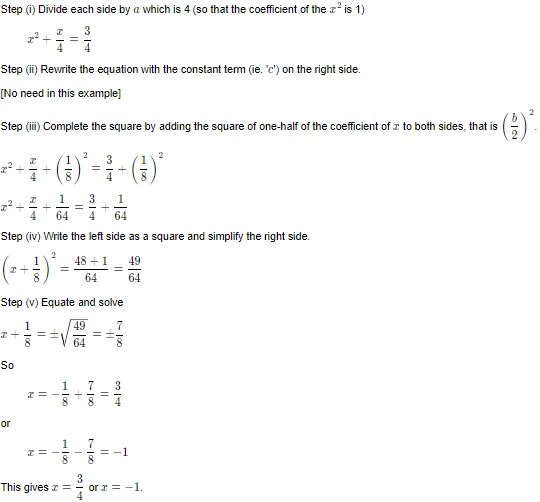In Graphing  Method One has to draw the Graph of given Quadratic Equation & the solution will appear on graph itself.

NOTE:

If the graph touches the X axis at two points, then it means that the given Quadratic equation has Two Distinct Real Roots. It will happen in the case where discriminant or D= b2 – 4ac > 0.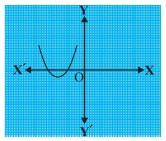If the graph touches the X axis at a single point, then it means that the given Quadratic equation has One or Unique Real Roots. It will happen in the case where discriminant or D= b2 – 4ac = 0.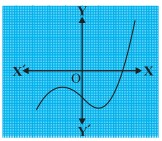If the graph Never touches the X axis at any point, then it means that the given Quadratic equation has No Real Roots. It will happen in the case where discriminant or D= b2 – 4ac < 0.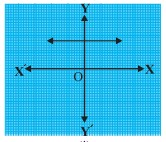For finding Roots of a Quadratic Equation by Using Quadratic Formula, one has to just apply formula.